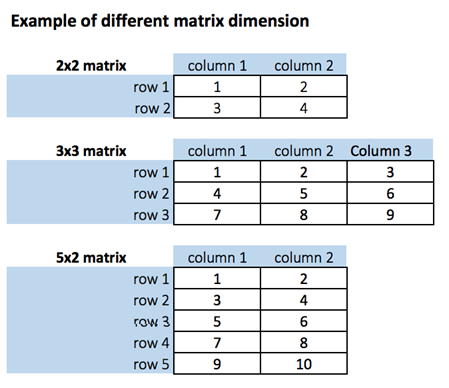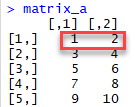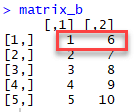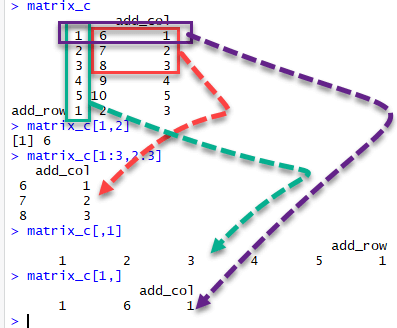# R Matrix Tutorial: Create, Print, add Column, Slice

## Matrix Function in R

A matrix function in R is a 2-dimensional array that has m number of rows and n number of columns. In other words, matrix in R programming is a combination of two or more vectors with the same data type.

Note: It is possible to create more than two dimensions arrays with matrix function in R.## How to Create a Matrix in R

We can create a matrix with the function matrix(). Following is a function to create a matrix in R which takes three arguments:

matrix(data, nrow, ncol, byrow = FALSE)

Arguments:

• data : The collection of elements that R will arrange into the rows and columns of the matrix \
• nrow : Number of rows
• ncol : Number of columns
• byrow : The rows are filled from the left to the right. We use `byrow = FALSE` (default values), if we want the matrix to be filled by the columns i.e. the values are filled top to bottom.

Let’s construct two 5x2 matrix with a sequence of number from 1 to 10, one with byrow = TRUE and one with byrow = FALSE to see the difference.

How to write a TEST CASE Software Testing Tutorial

# Construct a matrix with 5 rows that contain the numbers 1 up to 10 and byrow = TRUE matrix_a <-matrix(1:10, byrow = TRUE, nrow = 5) matrix_a

Output:#### Print dimension of the matrix with dim()

Now, let’s print dimension of the matrix in R with dim(). The syntax to print matrix in R using dim() is:

Output:

# Construct a matrix with 5 rows that contain the numbers 1 up to 10 and byrow = FALSE matrix_b <-matrix(1:10, byrow = FALSE, nrow = 5) matrix_b

Output:#### Print dimension of the matrix with dim()

Again, print the dimension of the matrix using dim(). Below is a syntax of R print matrix dimension:

# Print dimension of the matrix with dim() dim(matrix_b)

Output:

##  5 2

Note : Using command matrix_b <-matrix(1:10, byrow = FALSE, ncol = 2) will have same effect as above.

You can also create a 4x3 matrix using ncol. R will create 3 columns and fill the row from top to bottom. Check an example

matrix_c <-matrix(1:12, byrow = FALSE, ncol = 3) matrix_c

Output:

Example:

dim(matrix_c)

Output:

## Add a Column to a Matrix with the cbind()

You can add column to matrix R with the cbind() command. cbind() means column binding. cbind() can concatenate as many matrix or columns as specified. For example, our previous example created a 5x2 matrix. We concatenate a third column and verify the dimension is 5x3

Example:

# concatenate c(1:5) to the matrix_a matrix_a1 <- cbind(matrix_a, c(1:5)) # Check the dimension dim(matrix_a1)

Output:

Example:

matrix_a1

Output

## [,1] [,2] [,3] ## [1,] 1 2 1 ## [2,] 3 4 2 ## [3,] 5 6 3 ## [4,] 7 8 4 ## [5,] 9 10 5

Example:

We can also add column to matrix R, more than one time. Let’s see the next sequence of number to the matrix_a2 matrix. The dimension of new matrices in R will be 4x6 with number from 1 to 24.

matrix_a2 <-matrix(13:24, byrow = FALSE, ncol = 3)

Output:

## [,1] [,2] [,3] ## [1,] 13 17 21 ## [2,] 14 18 22 ## [3,] 15 19 23 ## [4,] 16 20 24

Example:

matrix_c <-matrix(1:12, byrow = FALSE, ncol = 3) matrix_d <- cbind(matrix_a2, matrix_c) dim(matrix_d)

Output:

##  4 6

NOTE : The number of rows of matrices in R should be equal for cbind work

cbind()concatenate columns, rbind() appends rows. Let’s add one row to our matrix_c matrix and verify the dimension is 5x3

matrix_c <-matrix(1:12, byrow = FALSE, ncol = 3) # Create a vector of 3 columns add_row <- c(1:3) # Append to the matrix matrix_c <- rbind(matrix_c, add_row) # Check the dimension dim(matrix_c)

Output:

## Slice a Matrix

We can select elements one or many elements from a matrix in R programming by using the square brackets [ ]. This is where slicing comes into the picture.

For example:

• matrix_c[1,2] selects the element at the first row and second column.
• matrix_c[1:3,2:3] results in a R slice matrix with the data on the rows 1, 2, 3 and columns 2, 3,
• matrix_c[,1] selects all elements of the first column.
• matrix_c[1,] selects all elements of the first row.

Here is the output you get for the above codes